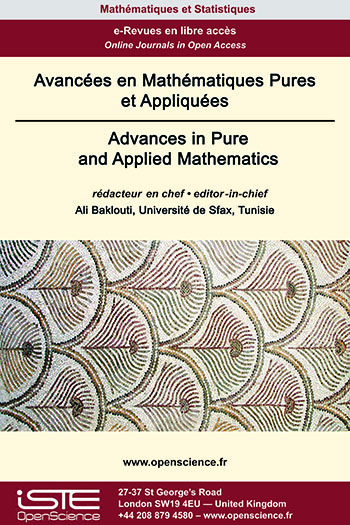# Vol 12 - Issue 4 (September 2021)

## List of Articles

Multiplicity of solutions for a nonhomogeneous problem involving a potential in Orlicz-Sobolev spaces

This paper is devoted to the study of the nonhomogeneous problem
$-div (a(|\nabla u|)\nabla u)+a(| u|)u=\lambda V(x)|u|^{m(x)-2}u-\mu g(x,u) \mbox{ in} \ \Omega, \ u=0 \mbox{ on} \ \partial\Omega ,$ where $\Omega$ is a bounded smooth domain in $\mathbb{R}^N,\lambda, \mu$ are positive real numbers, $V(x)$ is a potential, $m: \overline{ \Omega} \to (1, \infty)$ is a continuous function, $a$ is mapping such that $\varphi(|t|)t$ is increasing homeomorphism from ℝ to ℝ and $g: \overline{\Omega}\times ℝ \to ℝ$ is a continuous function. We establish there main results with various assumptions, the first one asserts that any $\lambda$0> is an eigenvalue of our problem. The second Theorem states the existence of a constant $\lambda^{*}$ such that every $\lambda \in (0,\lambda^{*})$ is an eigenvalue of the problem. While the third Theorem claims the existence of a constant $\lambda^{**}$ such that every $\lambda \in [\lambda^{**},\infty)$ is an eigenvalue of the problem. Our approach relies on adequate variational methods in Orlicz-Sobolev spaces.

General Decay Of A Nonlinear Viscoelastic Wave: Equation With Boundary Dissipation

In this work we establish a general decay rate for a nonlinear viscoelastic wave equation with boundary dissipation where the relaxation function satisfies $g^{\prime }\left( t\right) \leq -\xi \left( t\right) g^{p} % \left( t\right) , t\geq 0, 1\leq p\leq \frac{3}{2}.$ This work generalizes and improves earlier results in the literature.

Scattering theory in weighted L2 space for a class of the defocusing inhomogeneous nonlinear Schrödinger equation

In this paper, we consider the following inhomogeneous nonlinear Schrödinger equation (INLS)
$i\partial_t u + \Delta u + \mu$ |$x$|$^{-b}$|$u$|$^\alpha u = 0, \quad (t,x)\in ℝ \times ℝ^d$
with $b, \alpha$ > 0. First, we revisit the local well-posedness in $H^1(ℝ^d)$ for (INLS) of Guzmán [Nonlinear Anal. Real World Appl. 37 (2017), 249-286] and give an improvement of this result in the two and three spatial dimensional cases. Second, we study the decay of global solutions for the defocusing (INLS), i.e. $\mu=-1$ when 0 < $\alpha$ < $\alpha^\star$ where $\alpha^\star = \frac{4-2b}{d-2}$ for $d\geq 3$, and $\alpha^\star = \infty$ for $d=1, 2$
by assuming that the initial data belongs to the weighted $L^2$ space $\Sigma =\{u \in H^1(ℝ^d) :$ |$x$|$u \in L^2(ℝ^d) \}$. Finally, we combine the local theory and the decaying property to show the scattering in $\Sigma$ for the defocusing (INLS) in the case $\alpha_\star$ < $\alpha$ < $\alpha^\star$, where $\alpha_\star = \frac{4-2b}{d}$.

Exponential stability of discrete-time damped Schrödinger equation

This article concerns the discrete-time problem of the damped Schrödinger equation in a bounded domain and the internal stabilization problem is considered. We constructed a well-posed discrete-time scheme and we proved the exponential stability. Numerical simulations are presented to illustrate the theoretical results.### Other issues :

2020

Volume 20- 11

Issue 1 (May 2020)
Issue 2 (September 2020)

2021

Volume 21- 12

Issue 1 (January 2021)
Issue 2 (May 2021)
Issue 3 (Special AUS-ICMS 2020)
Issue 4 (September 2021)

2022

Volume 22- 13

Forthcoming papers

Issue 1 (January 2022)
Issue 2 (March 2022)
Issue 3 (June 2022)